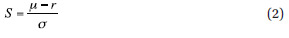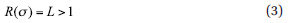Optimal Design Of Risk Control Strategy Indexes

August 17, 2012

Based on the index methodology (1), the objective of our research is to answer the following question: Assume the underlying equity index St has an average yearly return ?, an average volatility ? and consequently a long-run Sharpe ratiowith r denoting the average refinancing rate. Given these parameters of the underlying equity index, the question is whether it is possible to predict the long-run return and Sharpe ratio of the strategy index calculated according to formula (1) without knowing the entire path of the underlying equity index, i.e., only using the return and volatility of the underlying equity index and the response function R(?t).

We will show next that this is indeed possible for all existing types of strategy indexes based on the concept (1); in particular, leveraged indexes and target volatility indexes.

In a second step, we will use our understanding of the risk/ return characteristics of strategy indexes calculated according to the index formula (1) to derive optimally risk-controlled versions of leveraged indexes and target volatility indexes.

Analysis Of Existing Strategy Index Concepts
We start with an analysis of well-known investment strategies that are already available in the market in the form of strategy indexes and corresponding retail investment products: leveraged indexes and target-risk indexes.

Leveraged Indexes
The existing model of leveraged indexes has become quite popular in the ETF market in recent years (see EDHEC 2009). In fact, leveraged indexes are the simplest form of a strategy index using the index formula (1) in the sense that they are based on a constant response function. In the language of our model (1) we have (see NYSE Euronext 2008, Stoxx 2010):where most leveraged ETFs use a constant leverage of L=2. In essence, the investment strategy (3) does not respond to equity volatility at all, which means it is not risk controlled in any way, and later in this article we will use the results derived for this type of leveraged index to show the advantages of strategies that are risk controlled.

Leveraged indexes have been widely criticized because their performance depends on the path of the underlying, as the index formula (1) suggests, and therefore their performance characteristics are very complex.